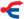关于学徒的用法，请见“黑爪”。

—— 黑掌对豹星，在森林大会上，午夜追踪》，第44页

Blackclaw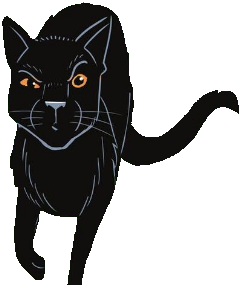《河族阴云》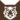虎族 (Q645)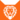狮族 (Q646)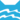河族 武士河族 长老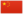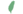小黑 Blackkit  小黑
 黑爪 Blackpaw  黑掌
 黑掌 Blackclaw  黑爪

Q2665：河族公猫，自《预言开始》系列登场的角色

## 细节

### 外貌

• 他是一只橘色眼睛的烟黑色公猫，:44身材精瘦:51且肌肉发达。:191他有着修长的四肢:51和尾巴:23，一只耳尖撕裂。:303

### 作者声明

• WarriorCats.com的家谱树中，他是雾星的伴侣，小鲈鱼、樱草爪、矛爪和芦苇须的父亲。

### 勘误

• 他曾被错误地描述为深灰色。:259
• 他曾被错误地描述为风族猫。:7
• 他曾被错误地当成黑星:30
• 在《钩星的承诺》中，为了不让小豹孤苦伶仃地留在育婴室中，包含他在内的其他幼崽都同意推迟学徒仪式；:337但在《豹星的荣耀》中，小豹却在五个月大时提早晋升为学徒。:2章

## 登场书目

• 寒冰烈火 (Q59)[配角][首次登场🐱]
• 疑云重重 (Q60)[配角]
• 风起云涌 (Q65)[猫物闲角]
• 险路惊魂 (Q66)[配角]
• 力挽狂澜 (Q67)[配角]
• 午夜追踪 (Q139)[配角]
• 新月危机 (Q140)[猫物闲角]
• 重现家园 (Q141)[猫物闲角]
• 星光指路 (Q142)[配角]
• 黄昏战争 (Q143)[配角]
• 日落和平 (Q144)[配角]
• 预视力量 (Q152)[猫物闲角]
• 暗河汹涌 (Q153)[配角]
• 驱逐之战 (Q154)[猫物闲角]
• 天蚀遮月 (Q155)[猫物闲角]
• 暗夜长影 (Q156)[猫物闲角]
• 拂晓之光 (Q157)[猫物闲角]
• 第四学徒 (Q158)[配角]
• 战声渐近 (Q159)[对话提及][死亡时刻✝]
• 火星的探索 (Q176)[猫物闲角]
• 钩星的承诺 (Q179)[配角]
• 灰条的誓言 (Q4057)[猫物闲角]
• 豹星的荣耀 (Q4426)[配角]
• 冬青叶的故事 (Q187)[猫物闲角]
• 雾星的征兆 (Q188)[配角]
• 虎掌的愤怒 (Q190)[猫物闲角]
• 叶池的祈愿 (Q191)[猫物闲角]
• 红尾的恩债 (Q3565)[猫物闲角]
• 蛾翅的秘密 (Q3628)[配角]
• 黑脚的清算 (Q4336)[猫物闲角]
• 返回族群 (Q212)[配角]
• 河族阴云 (Q4311)[配角]
• 变革之风 (Q4411)[配角]
• 族群的战争 (Q202)[配角]
• 族群决定 (Q4580)[配角]

## 参考文献

这篇文章基于CC BY-SA 3.0许可使用了猫武士维基（英语）Blackclaw一文中的部分内容。
0.0
0人评价
Feuerpfote
2

别看黑掌瘦，一身疙瘩肉【滑稽

1年